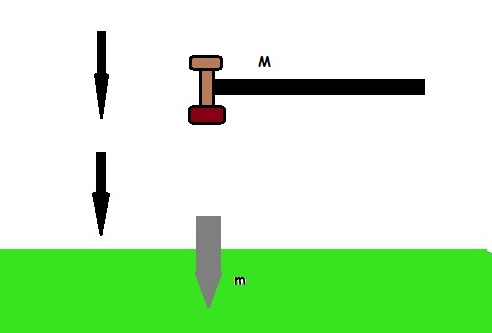# Strike it hardA hammer of mass $M$ falls from a height $l$ to strike a pile of mass $m$ into ground. Pile penetrates a distance $d$ in a single blow. Find the resistance to penetration given by ground in $Newtons$

Details and Assumptions

• Assume collision to be inelastic.
• Assume both the masses to be point masses.
• $M=0.5 \text{kg} , \space m=5\text{kg} , \space l=22\text{cm}, \space d = 5\text{cm} , \space g=\SI[per-mode=symbol]{10}{\meter\per\second\squared}.$
×

Problem Loading...

Note Loading...

Set Loading...